Home > A2C > Chapter 9 > Lesson 9.1.2 > Problem9-43

9-43.
1. A sequence of pentagonal numbers is started below. Homework Help ✎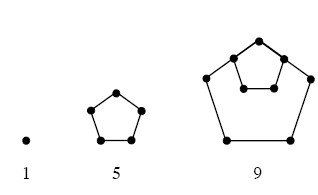1. Find the next three pentagonal numbers.

2. What kind of sequence do the pentagonal numbers form?

3. What is the equation for the nth pentagonal number?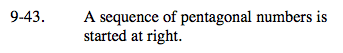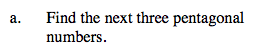13, 17, 21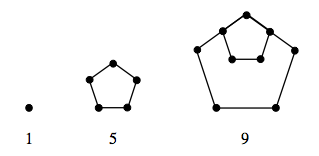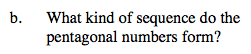Is a constant being added to each number to obtain the next? Multiplied? Neither?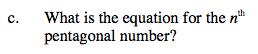t(n) = 4n − 3